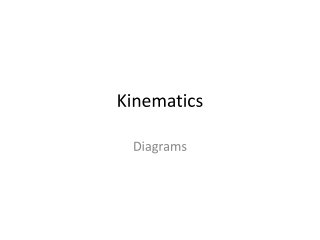DownloadDownload PresentationKinematics

# Kinematics

Télécharger la présentation## Kinematics

- - - - - - - - - - - - - - - - - - - - - - - - - - - E N D - - - - - - - - - - - - - - - - - - - - - - - - - - -
##### Presentation Transcript

1. Kinematics Diagrams

2. Our topics today: • Describing Motion with Diagrams • Stroboscopic Images • Vector Diagrams • x-t, v-t, a-t graphs • Motion in constant acceleration • 1D Kinematic Equations • That’s it, folks!

3. Stroboscopic Images time 1 sec 2 sec 3 sec position 1 cm 5 cm 11 cm

4. Stroboscopic Images

5. Stroboscopic Images

6. Stroboscopic Images

7. Stroboscopic Images

8. Stroboscopic images • Try daw ang interactive physics. ;)

9. Vector Diagrams Using Displacement Vectors

10. Displacement-time (x-t) graphs

11. Displacement-time (x-t) graphs

12. Displacement-time (x-t) graphs

13. Displacement-time (x-t) graphs

14. Displacement-time (x-t) graphs • Which is which? • Positive and changing velocity (acceleration) • Positive and constant velocity A B Get 1/4

15. Displacement-time (x-t) graphs Which is which? 3. Slow, leftward (-), Constant Velocity 4. Fast, leftward (-), Constant velocity 5. Slow, rightward (+), constant velocity 6. Fast, rightward (+), constant velocity A B C D

16. Displacement-time (x-t) graphs Which is which? 7. Negative velocity (-), slow to fast 8. Leftward velocity (-), fast to slow A B

17. Velocity-time (v-t) graphs Constant Velocity Changing Velocity, Constant Acceleration

18. Velocity-time (v-t) graphs

19. Velocity-time (v-t) graphs

20. Velocity-time (v-t) graphs The area bounded by the “curve” and the x-axis determines the displacement, or how far the ball travels.

21. Motion in Constant Acceleration • That’s something for the black board.  • Reminder: Quiz Next Meeting • Vectors • Vector properties • Resolving vectors into x- and y- components • Numerical addition of vectors • Et cetera